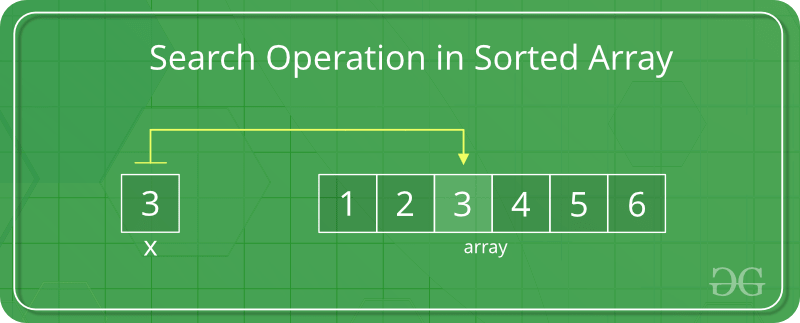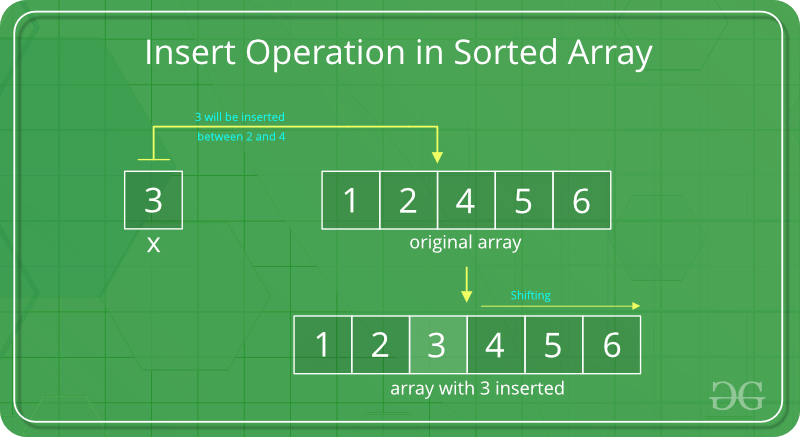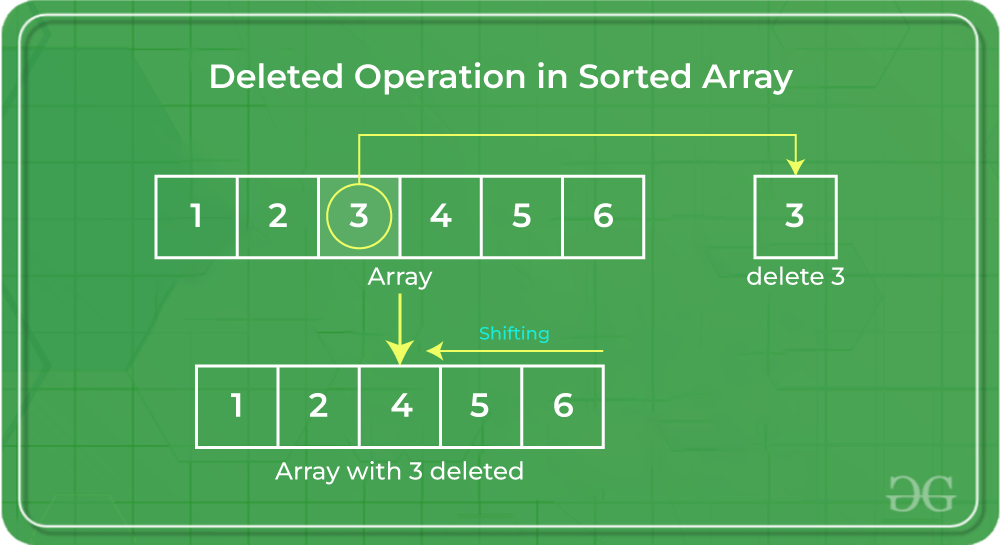GeeksforGeeks App
Open AppBrowser
Continue

# Search, insert and delete in a sorted array

## How to Search in a Sorted Array?

In a sorted array, the search operation can be performed by using binary search.Below is the implementation of the above approach:

## C++

 `// C++ program to implement binary search in sorted array``#include ``using` `namespace` `std;` `int` `binarySearch(``int` `arr[], ``int` `low, ``int` `high, ``int` `key)``{``    ``if` `(high < low)``        ``return` `-1;``    ``int` `mid = (low + high) / 2; ``/*low + (high - low)/2;*/``    ``if` `(key == arr[mid])``        ``return` `mid;``    ``if` `(key > arr[mid])``        ``return` `binarySearch(arr, (mid + 1), high, key);``    ``return` `binarySearch(arr, low, (mid - 1), key);``}` `/* Driver code */``int` `main()``{``    ``// Let us search 3 in below array``    ``int` `arr[] = { 5, 6, 7, 8, 9, 10 };``    ``int` `n, key;` `    ``n = ``sizeof``(arr) / ``sizeof``(arr);``    ``key = 10;` `    ``// Function call``    ``cout << ``"Index: "` `<< binarySearch(arr, 0, n - 1, key)``         ``<< endl;``    ``return` `0;``}` `// This code is contributed by NamrataSrivastava1`

## C

 `// C program to implement binary search in sorted array``#include ` `int` `binarySearch(``int` `arr[], ``int` `low, ``int` `high, ``int` `key)``{``    ``if` `(high < low)``        ``return` `-1;``    ``int` `mid = (low + high) / 2; ``/*low + (high - low)/2;*/``    ``if` `(key == arr[mid])``        ``return` `mid;``    ``if` `(key > arr[mid])``        ``return` `binarySearch(arr, (mid + 1), high, key);``    ``return` `binarySearch(arr, low, (mid - 1), key);``}` `/* Driver Code */``int` `main()``{``    ``// Let us search 3 in below array``    ``int` `arr[] = { 5, 6, 7, 8, 9, 10 };``    ``int` `n, key;` `    ``n = ``sizeof``(arr) / ``sizeof``(arr);``    ``key = 10;` `    ``// Function call``    ``printf``(``"Index: %d\n"``, binarySearch(arr, 0, n - 1, key));``    ``return` `0;``}`

## Java

 `// Java program to implement binary``// search in a sorted array` `class` `Main {``    ``// function to implement``    ``// binary search``    ``static` `int` `binarySearch(``int` `arr[], ``int` `low, ``int` `high,``                            ``int` `key)``    ``{``        ``if` `(high < low)``            ``return` `-``1``;` `        ``/*low + (high - low)/2;*/``        ``int` `mid = (low + high) / ``2``;``        ``if` `(key == arr[mid])``            ``return` `mid;``        ``if` `(key > arr[mid])``            ``return` `binarySearch(arr, (mid + ``1``), high, key);``        ``return` `binarySearch(arr, low, (mid - ``1``), key);``    ``}` `    ``/* Driver Code*/``    ``public` `static` `void` `main(String[] args)``    ``{``        ``int` `arr[] = { ``5``, ``6``, ``7``, ``8``, ``9``, ``10` `};``        ``int` `n, key;``        ``n = arr.length - ``1``;``        ``key = ``10``;` `        ``// Function call``        ``System.out.println(``"Index: "``                           ``+ binarySearch(arr, ``0``, n, key));``    ``}``}`

## Python3

 `# python 3  program to implement``# binary search in sorted array`  `def` `binarySearch(arr, low, high, key):` `    ``mid ``=` `(low ``+` `high)``/``2` `    ``if` `(key ``=``=` `arr[``int``(mid)]):``        ``return` `mid` `    ``if` `(key > arr[``int``(mid)]):``        ``return` `binarySearch(arr,``                            ``(mid ``+` `1``), high, key)` `    ``if` `(key < arr[``int``(mid)]):``        ``return` `binarySearch(arr, low, (mid``-``1``), key)` `    ``return` `0`  `# Driver code``if` `__name__ ``=``=` `"__main__"``:``    ``# Let us search 3 in below array``    ``arr ``=` `[``5``, ``6``, ``7``, ``8``, ``9``, ``10``]``    ``n ``=` `len``(arr)``    ``key ``=` `10` `    ``# Function call``    ``print``(``"Index:"``, ``int``(binarySearch(arr, ``0``, n``-``1``, key)))` `# This code is contributed by``# Smitha Dinesh Semwal`

## C#

 `// C# program to implement binary``// search in a sorted array` `using` `System;` `public` `class` `GFG {` `    ``// function to implement``    ``// binary search``    ``public` `static` `int` `binarySearch(``int``[] arr, ``int` `low,``                                   ``int` `high, ``int` `key)``    ``{``        ``if` `(high < low) {``            ``return` `-1;``        ``}` `        ``int` `mid = (low + high) / 2;``        ``if` `(key == arr[mid]) {``            ``return` `mid;``        ``}``        ``if` `(key > arr[mid]) {``            ``return` `binarySearch(arr, (mid + 1), high, key);``        ``}``        ``return` `binarySearch(arr, low, (mid - 1), key);``    ``}` `    ``/* Driver Code */``    ``public` `static` `void` `Main(``string``[] args)``    ``{``        ``int``[] arr = ``new` `int``[] { 5, 6, 7, 8, 9, 10 };``        ``int` `n, key;``        ``n = arr.Length;``        ``key = 10;` `        ``// Function call``        ``Console.WriteLine(``            ``"Index: "` `+ binarySearch(arr, 0, n - 1, key));``    ``}``}` `// This code is contributed by Shrikant13`

## PHP

 ` ``\$arr``[(int)``\$mid``])``        ``return` `binarySearch(``\$arr``, (``\$mid` `+ 1),``                            ``\$high``, ``\$key``);``    ` `    ``return` `(binarySearch(``\$arr``, ``\$low``,``                        ``(``\$mid` `-1), ``\$key``));``}` `// Driver Code` `// Let us search 3 in below array``\$arr` `= ``array``(5, 6, 7, 8, 9, 10);``\$n` `= ``count``(``\$arr``);``\$key` `= 10;` `// Function call``echo` `"Index: "``, (int)binarySearch(``\$arr``, 0,``                                  ``\$n``-1, ``\$key``);` `// This code is contributed by``// Srathore``?>`

## Javascript

 ``

Output

`Index: 5`

Time Complexity: O(log(n)) Using Binary Search
Auxiliary Space: O(log(n)) due to recursive calls, otherwise iterative version uses Auxiliary Space of O(1).

## How to Insert in a Sorted Array?

In a sorted array, a search operation is performed for the possible position of the given element by using Binary search, and then an insert operation is performed followed by shifting the elements. And in an unsorted array, the insert operation is faster as compared to the sorted array because we don’t have to care about the position at which the element is placed.Below is the implementation of the above approach:

## C++

 `// C++ program to implement insert operation in``// an sorted array.``#include ``using` `namespace` `std;` `// Inserts a key in arr[] of given capacity. n is current``// size of arr[]. This function returns n+1 if insertion``// is successful, else n.``int` `insertSorted(``int` `arr[], ``int` `n, ``int` `key, ``int` `capacity)``{``    ``// Cannot insert more elements if n is already``    ``// more than or equal to capacity``    ``if` `(n >= capacity)``        ``return` `n;` `    ``int` `i;``    ``for` `(i = n - 1; (i >= 0 && arr[i] > key); i--)``        ``arr[i + 1] = arr[i];` `    ``arr[i + 1] = key;` `    ``return` `(n + 1);``}` `/* Driver code */``int` `main()``{``    ``int` `arr = { 12, 16, 20, 40, 50, 70 };``    ``int` `capacity = ``sizeof``(arr) / ``sizeof``(arr);``    ``int` `n = 6;``    ``int` `i, key = 26;` `    ``cout << ``"\nBefore Insertion: "``;``    ``for` `(i = 0; i < n; i++)``        ``cout << arr[i] << ``" "``;` `    ``// Function call``    ``n = insertSorted(arr, n, key, capacity);` `    ``cout << ``"\nAfter Insertion: "``;``    ``for` `(i = 0; i < n; i++)``        ``cout << arr[i] << ``" "``;` `    ``return` `0;``}` `// This code is contributed by SHUBHAMSINGH10`

## C

 `// C program to implement insert operation in``// an sorted array.``#include ` `// Inserts a key in arr[] of given capacity.  n is current``// size of arr[]. This function returns n+1 if insertion``// is successful, else n.``int` `insertSorted(``int` `arr[], ``int` `n, ``int` `key, ``int` `capacity)``{``    ``// Cannot insert more elements if n is already``    ``// more than or equal to capacity``    ``if` `(n >= capacity)``        ``return` `n;` `    ``int` `i;``    ``for` `(i = n - 1; (i >= 0 && arr[i] > key); i--)``        ``arr[i + 1] = arr[i];` `    ``arr[i + 1] = key;` `    ``return` `(n + 1);``}` `/* Driver code */``int` `main()``{``    ``int` `arr = { 12, 16, 20, 40, 50, 70 };``    ``int` `capacity = ``sizeof``(arr) / ``sizeof``(arr);``    ``int` `n = 6;``    ``int` `i, key = 26;` `    ``printf``(``"\nBefore Insertion: "``);``    ``for` `(i = 0; i < n; i++)``        ``printf``(``"%d  "``, arr[i]);` `    ``// Function call``    ``n = insertSorted(arr, n, key, capacity);` `    ``printf``(``"\nAfter Insertion: "``);``    ``for` `(i = 0; i < n; i++)``        ``printf``(``"%d  "``, arr[i]);` `    ``return` `0;``}`

## Java

 `// Java program to insert an``// element in a sorted array` `class` `Main {``    ``// Inserts a key in arr[] of given``    ``// capacity.  n is current size of arr[].``    ``// This function returns n+1 if insertion``    ``// is successful, else n.``    ``static` `int` `insertSorted(``int` `arr[], ``int` `n, ``int` `key,``                            ``int` `capacity)``    ``{``        ``// Cannot insert more elements if n is already``        ``// more than or equal to capacity``        ``if` `(n >= capacity)``            ``return` `n;` `        ``int` `i;``        ``for` `(i = n - ``1``; (i >= ``0` `&& arr[i] > key); i--)``            ``arr[i + ``1``] = arr[i];` `        ``arr[i + ``1``] = key;` `        ``return` `(n + ``1``);``    ``}` `    ``/* Driver code */``    ``public` `static` `void` `main(String[] args)``    ``{``        ``int` `arr[] = ``new` `int``[``20``];``        ``arr[``0``] = ``12``;``        ``arr[``1``] = ``16``;``        ``arr[``2``] = ``20``;``        ``arr[``3``] = ``40``;``        ``arr[``4``] = ``50``;``        ``arr[``5``] = ``70``;``        ``int` `capacity = arr.length;``        ``int` `n = ``6``;``        ``int` `key = ``26``;` `        ``System.out.print(``"\nBefore Insertion: "``);``        ``for` `(``int` `i = ``0``; i < n; i++)``            ``System.out.print(arr[i] + ``" "``);` `        ``// Function call``        ``n = insertSorted(arr, n, key, capacity);` `        ``System.out.print(``"\nAfter Insertion: "``);``        ``for` `(``int` `i = ``0``; i < n; i++)``            ``System.out.print(arr[i] + ``" "``);``    ``}``}`

## Python3

 `# Python3 program to implement insert``# operation in an sorted array.` `# Inserts a key in arr[] of given capacity.``# n is current size of arr[]. This function``# returns n+1 if insertion is successful, else n.`  `def` `insertSorted(arr, n, key, capacity):` `    ``# Cannot insert more elements if n is``    ``# already more than or equal to capacity``    ``if` `(n >``=` `capacity):``        ``return` `n` `    ``i ``=` `n ``-` `1``    ``while` `i >``=` `0` `and` `arr[i] > key:``        ``arr[i ``+` `1``] ``=` `arr[i]``        ``i ``-``=` `1` `    ``arr[i ``+` `1``] ``=` `key` `    ``return` `(n ``+` `1``)`  `# Driver Code``if` `__name__ ``=``=` `"__main__"``:``    ``arr ``=` `[``12``, ``16``, ``20``, ``40``, ``50``, ``70``]` `    ``for` `i ``in` `range``(``20``):``        ``arr.append(``0``)` `    ``capacity ``=` `len``(arr)``    ``n ``=` `6``    ``key ``=` `26` `    ``print``(``"Before Insertion: "``, end``=``" "``)``    ``for` `i ``in` `range``(n):``        ``print``(arr[i], end``=``" "``)` `    ``# Function call``    ``n ``=` `insertSorted(arr, n, key, capacity)` `    ``print``(``"\nAfter Insertion: "``, end``=``"")``    ``for` `i ``in` `range``(n):``        ``print``(arr[i], end``=``" "``)` `# This code is contributed by Mohit Kumar`

## C#

 `using` `System;` `// C# program to insert an``// element in a sorted array` `public` `class` `GFG {` `    ``// Inserts a key in arr[] of given``    ``// capacity.  n is current size of arr[].``    ``// This function returns n+1 if insertion``    ``// is successful, else n.``    ``public` `static` `int` `insertSorted(``int``[] arr, ``int` `n,``                                   ``int` `key, ``int` `capacity)``    ``{``        ``// Cannot insert more elements if n is already``        ``// more than or equal to capacity``        ``if` `(n >= capacity) {``            ``return` `n;``        ``}` `        ``int` `i;``        ``for` `(i = n - 1; (i >= 0 && arr[i] > key); i--) {``            ``arr[i + 1] = arr[i];``        ``}` `        ``arr[i + 1] = key;` `        ``return` `(n + 1);``    ``}` `    ``/* Driver code */``    ``public` `static` `void` `Main(``string``[] args)``    ``{``        ``int``[] arr = ``new` `int``;``        ``arr = 12;``        ``arr = 16;``        ``arr = 20;``        ``arr = 40;``        ``arr = 50;``        ``arr = 70;``        ``int` `capacity = arr.Length;``        ``int` `n = 6;``        ``int` `key = 26;` `        ``Console.Write(``"\nBefore Insertion: "``);``        ``for` `(``int` `i = 0; i < n; i++) {``            ``Console.Write(arr[i] + ``" "``);``        ``}` `        ``// Function call``        ``n = insertSorted(arr, n, key, capacity);` `        ``Console.Write(``"\nAfter Insertion: "``);``        ``for` `(``int` `i = 0; i < n; i++) {``            ``Console.Write(arr[i] + ``" "``);``        ``}``    ``}``}` `// This code is contributed by Shrikant13`

## Javascript

 ``

Output

```Before Insertion: 12 16 20 40 50 70
After Insertion: 12 16 20 26 40 50 70 ```

Time Complexity: O(N) [In the worst case all elements may have to be moved]
Auxiliary Space: O(1)

## How to Delete in a Sorted Array?

In the delete operation, the element to be deleted is searched using binary search, and then the delete operation is performed followed by shifting the elements.Performing delete operation

Below is the implementation of the above approach:

## C++

 `// C++ program to implement delete operation in a``// sorted array``#include ``using` `namespace` `std;` `// To search a key to be deleted``int` `binarySearch(``int` `arr[], ``int` `low, ``int` `high, ``int` `key);` `/* Function to delete an element */``int` `deleteElement(``int` `arr[], ``int` `n, ``int` `key)``{``    ``// Find position of element to be deleted``    ``int` `pos = binarySearch(arr, 0, n - 1, key);` `    ``if` `(pos == -1) {``        ``cout << ``"Element not found"``;``        ``return` `n;``    ``}` `    ``// Deleting element``    ``int` `i;``    ``for` `(i = pos; i < n - 1; i++)``        ``arr[i] = arr[i + 1];` `    ``return` `n - 1;``}` `int` `binarySearch(``int` `arr[], ``int` `low, ``int` `high, ``int` `key)``{``    ``if` `(high < low)``        ``return` `-1;``    ``int` `mid = (low + high) / 2;``    ``if` `(key == arr[mid])``        ``return` `mid;``    ``if` `(key > arr[mid])``        ``return` `binarySearch(arr, (mid + 1), high, key);``    ``return` `binarySearch(arr, low, (mid - 1), key);``}` `// Driver code``int` `main()``{``    ``int` `i;``    ``int` `arr[] = { 10, 20, 30, 40, 50 };` `    ``int` `n = ``sizeof``(arr) / ``sizeof``(arr);``    ``int` `key = 30;` `    ``cout << ``"Array before deletion\n"``;``    ``for` `(i = 0; i < n; i++)``        ``cout << arr[i] << ``" "``;` `    ``// Function call``    ``n = deleteElement(arr, n, key);` `    ``cout << ``"\n\nArray after deletion\n"``;``    ``for` `(i = 0; i < n; i++)``        ``cout << arr[i] << ``" "``;``}` `// This code is contributed by shubhamsingh10`

## C

 `// C program to implement delete operation in a``// sorted array``#include ` `// To search a key to be deleted``int` `binarySearch(``int` `arr[], ``int` `low, ``int` `high, ``int` `key);` `/* Function to delete an element */``int` `deleteElement(``int` `arr[], ``int` `n, ``int` `key)``{``    ``// Find position of element to be deleted``    ``int` `pos = binarySearch(arr, 0, n - 1, key);` `    ``if` `(pos == -1) {``        ``printf``(``"Element not found"``);``        ``return` `n;``    ``}` `    ``// Deleting element``    ``int` `i;``    ``for` `(i = pos; i < n - 1; i++)``        ``arr[i] = arr[i + 1];` `    ``return` `n - 1;``}` `int` `binarySearch(``int` `arr[], ``int` `low, ``int` `high, ``int` `key)``{``    ``if` `(high < low)``        ``return` `-1;``    ``int` `mid = (low + high) / 2;``    ``if` `(key == arr[mid])``        ``return` `mid;``    ``if` `(key > arr[mid])``        ``return` `binarySearch(arr, (mid + 1), high, key);``    ``return` `binarySearch(arr, low, (mid - 1), key);``}` `// Driver code``int` `main()``{``    ``int` `i;``    ``int` `arr[] = { 10, 20, 30, 40, 50 };` `    ``int` `n = ``sizeof``(arr) / ``sizeof``(arr);``    ``int` `key = 30;` `    ``printf``(``"Array before deletion\n"``);``    ``for` `(i = 0; i < n; i++)``        ``printf``(``"%d  "``, arr[i]);` `    ``// Function call``    ``n = deleteElement(arr, n, key);` `    ``printf``(``"\n\nArray after deletion\n"``);``    ``for` `(i = 0; i < n; i++)``        ``printf``(``"%d  "``, arr[i]);``}`

## Java

 `// Java program to delete an``// element from a sorted array` `class` `Main {` `    ``// Binary search``    ``static` `int` `binarySearch(``int` `arr[], ``int` `low, ``int` `high,``                            ``int` `key)``    ``{``        ``if` `(high < low)``            ``return` `-``1``;``        ``int` `mid = (low + high) / ``2``;``        ``if` `(key == arr[mid])``            ``return` `mid;``        ``if` `(key > arr[mid])``            ``return` `binarySearch(arr, (mid + ``1``), high, key);``        ``return` `binarySearch(arr, low, (mid - ``1``), key);``    ``}` `    ``/* Function to delete an element */``    ``static` `int` `deleteElement(``int` `arr[], ``int` `n, ``int` `key)``    ``{``        ``// Find position of element to be deleted``        ``int` `pos = binarySearch(arr, ``0``, n - ``1``, key);` `        ``if` `(pos == -``1``) {``            ``System.out.println(``"Element not found"``);``            ``return` `n;``        ``}` `        ``// Deleting element``        ``int` `i;``        ``for` `(i = pos; i < n - ``1``; i++)``            ``arr[i] = arr[i + ``1``];` `        ``return` `n - ``1``;``    ``}` `    ``/* Driver Code */``    ``public` `static` `void` `main(String[] args)``    ``{` `        ``int` `i;``        ``int` `arr[] = { ``10``, ``20``, ``30``, ``40``, ``50` `};` `        ``int` `n = arr.length;``        ``int` `key = ``30``;` `        ``System.out.print(``"Array before deletion:\n"``);``        ``for` `(i = ``0``; i < n; i++)``            ``System.out.print(arr[i] + ``" "``);` `        ``// Function call``        ``n = deleteElement(arr, n, key);` `        ``System.out.print(``"\n\nArray after deletion:\n"``);``        ``for` `(i = ``0``; i < n; i++)``            ``System.out.print(arr[i] + ``" "``);``    ``}``}`

## Python3

 `# Python program to implement delete operation in a``# sorted array` `# /* Function to delete an element */`  `def` `deleteElement(arr, n, key):` `    ``# Find position of element to be deleted``    ``pos ``=` `binarySearch(arr, ``0``, n ``-` `1``, key)` `    ``if` `(pos ``=``=` `-``1``):``        ``print``(``"Element not found"``)``        ``return` `n` `    ``# Deleting element``    ``for` `i ``in` `range``(pos, n ``-` `1``):``        ``arr[i] ``=` `arr[i ``+` `1``]` `    ``return` `n ``-` `1` `# To search a key to be deleted`  `def` `binarySearch(arr, low, high, key):` `    ``if` `(high < low):``        ``return` `-``1``    ``mid ``=` `(low ``+` `high) ``/``/` `2` `    ``if` `(key ``=``=` `arr[mid]):``        ``return` `mid``    ``if` `(key > arr[mid]):``        ``return` `binarySearch(arr, (mid ``+` `1``), high, key)` `    ``return` `binarySearch(arr, low, (mid ``-` `1``), key)`  `# Driver code``if` `__name__ ``=``=` `"__main__"``:``    ``arr ``=` `[``10``, ``20``, ``30``, ``40``, ``50``]` `    ``n ``=` `len``(arr)``    ``key ``=` `30` `    ``print``(``"Array before deletion"``)` `    ``for` `i ``in` `range``(n):``        ``print``(arr[i], end``=``" "``)` `    ``# Function call``    ``n ``=` `deleteElement(arr, n, key)``    ``print``(``"\n\nArray after deletion"``)``    ``for` `i ``in` `range``(n):``        ``print``(arr[i], end``=``" "``)` `# This code is contributed by shubhamsingh10`

## C#

 `// C# program to delete an``// element from a sorted array``using` `System;``public` `class` `GFG {` `    ``// Binary search``    ``static` `int` `binarySearch(``int``[] arr, ``int` `low, ``int` `high,``                            ``int` `key)``    ``{``        ``if` `(high < low)``            ``return` `-1;``        ``int` `mid = (low + high) / 2;``        ``if` `(key == arr[mid])``            ``return` `mid;``        ``if` `(key > arr[mid])``            ``return` `binarySearch(arr, (mid + 1), high, key);``        ``return` `binarySearch(arr, low, (mid - 1), key);``    ``}` `    ``/* Function to delete an element */``    ``static` `int` `deleteElement(``int``[] arr, ``int` `n, ``int` `key)``    ``{``        ``// Find position of element to be deleted``        ``int` `pos = binarySearch(arr, 0, n - 1, key);` `        ``if` `(pos == -1) {``            ``Console.WriteLine(``"Element not found"``);``            ``return` `n;``        ``}` `        ``// Deleting element``        ``int` `i;``        ``for` `(i = pos; i < n - 1; i++)``            ``arr[i] = arr[i + 1];` `        ``return` `n - 1;``    ``}` `    ``/* Driver Code */``    ``public` `static` `void` `Main()``    ``{` `        ``int` `i;``        ``int``[] arr = { 10, 20, 30, 40, 50 };` `        ``int` `n = arr.Length;``        ``int` `key = 30;` `        ``Console.Write(``"Array before deletion:\n"``);``        ``for` `(i = 0; i < n; i++)``            ``Console.Write(arr[i] + ``" "``);` `        ``// Function call``        ``n = deleteElement(arr, n, key);` `        ``Console.Write(``"\n\nArray after deletion:\n"``);``        ``for` `(i = 0; i < n; i++)``            ``Console.Write(arr[i] + ``" "``);``    ``}``}` `// This code is contributed by Rajput-Ji`

## Javascript

 ``

Output

```Array before deletion
10 20 30 40 50

Array after deletion
10 20 40 50 ```

Time Complexity: O(N). In the worst case all elements may have to be moved
Auxiliary Space: O(log N). An implicit stack will be used

If you like GeeksforGeeks and would like to contribute, you can also write an article and mail your article to review-team@geeksforgeeks.org. See your article appearing on the GeeksforGeeks main page and help other Geeks. Please write comments if you find anything incorrect, or if you want to share more information about the topic discussed above

My Personal Notes arrow_drop_up